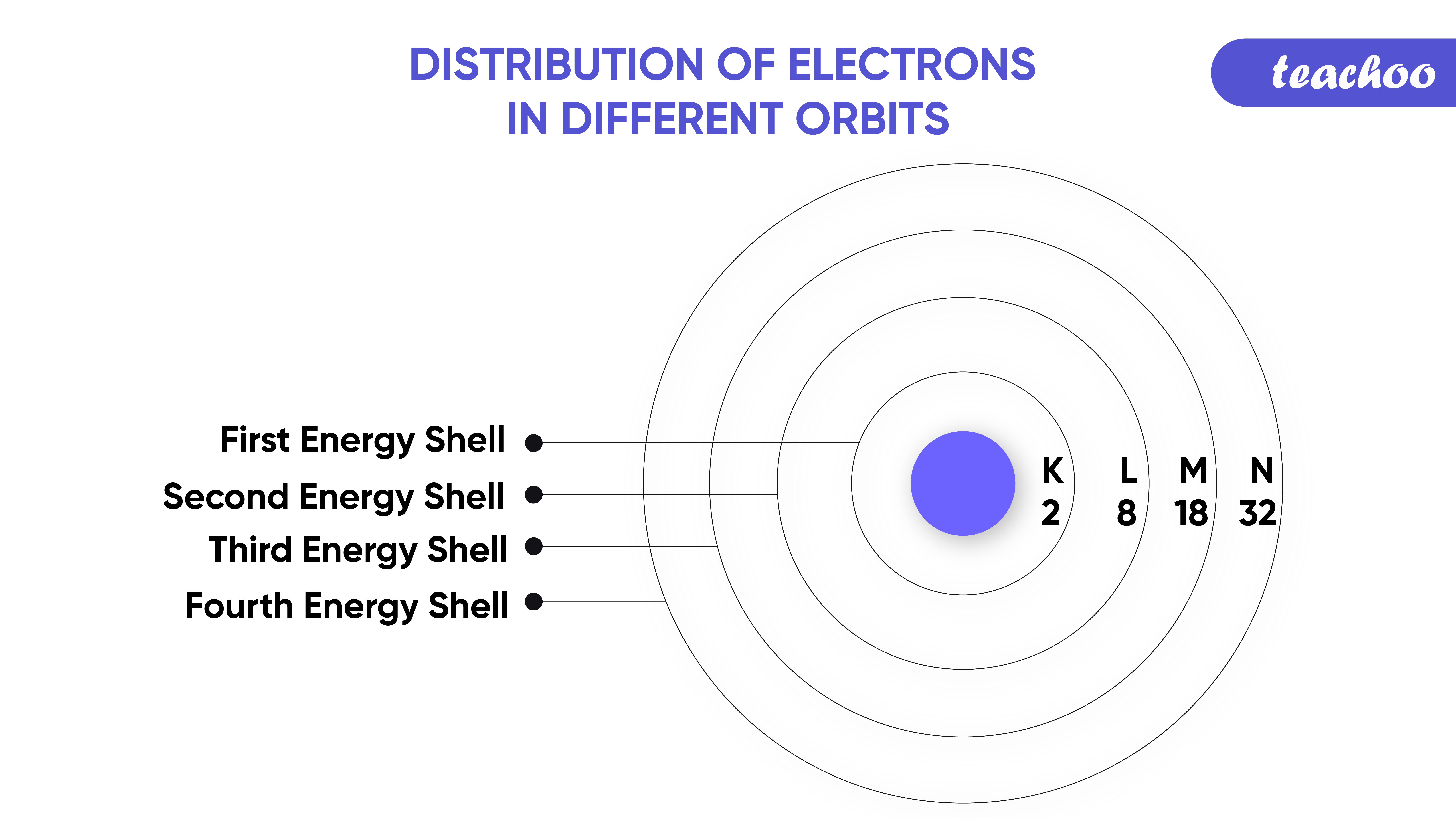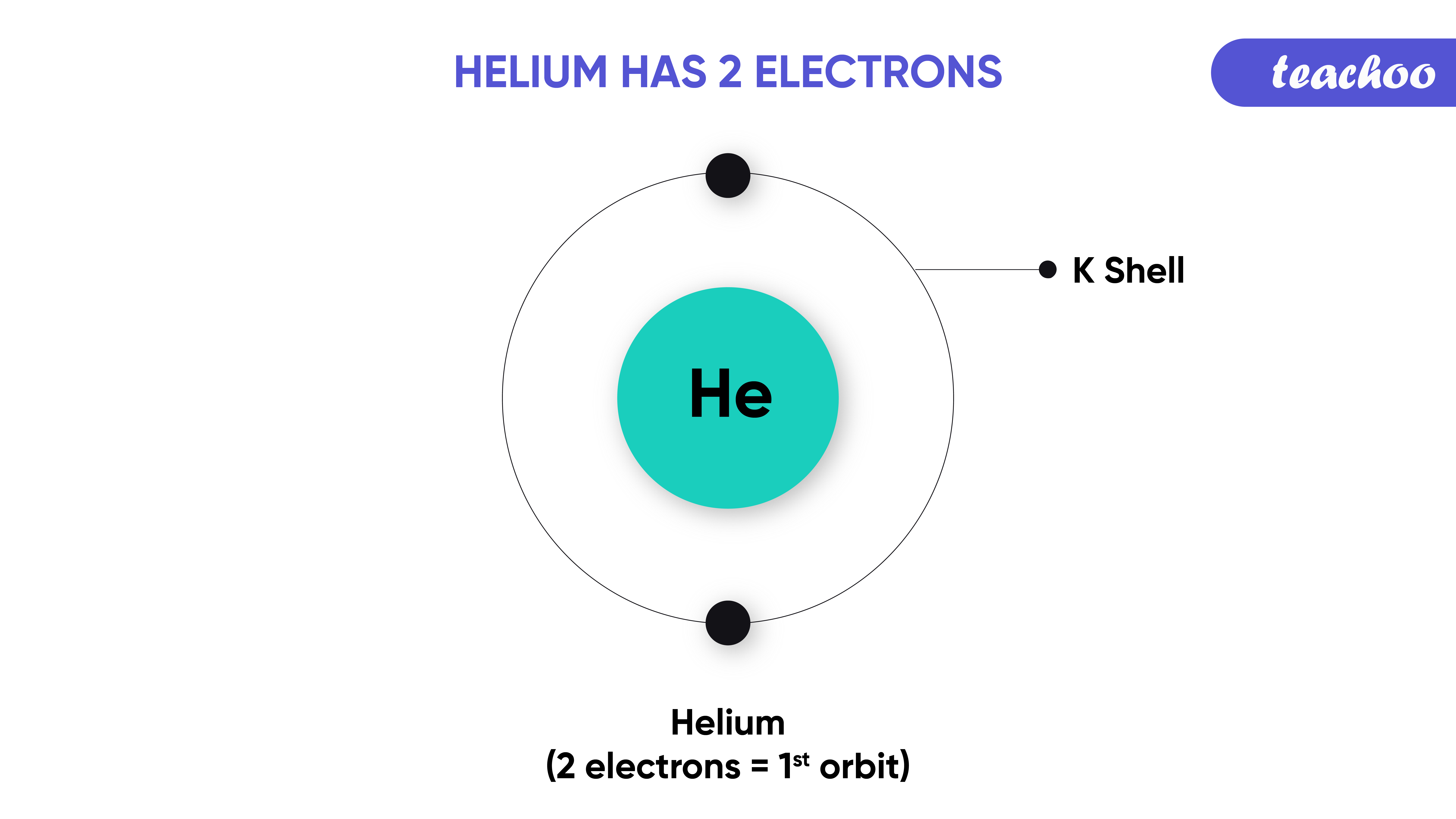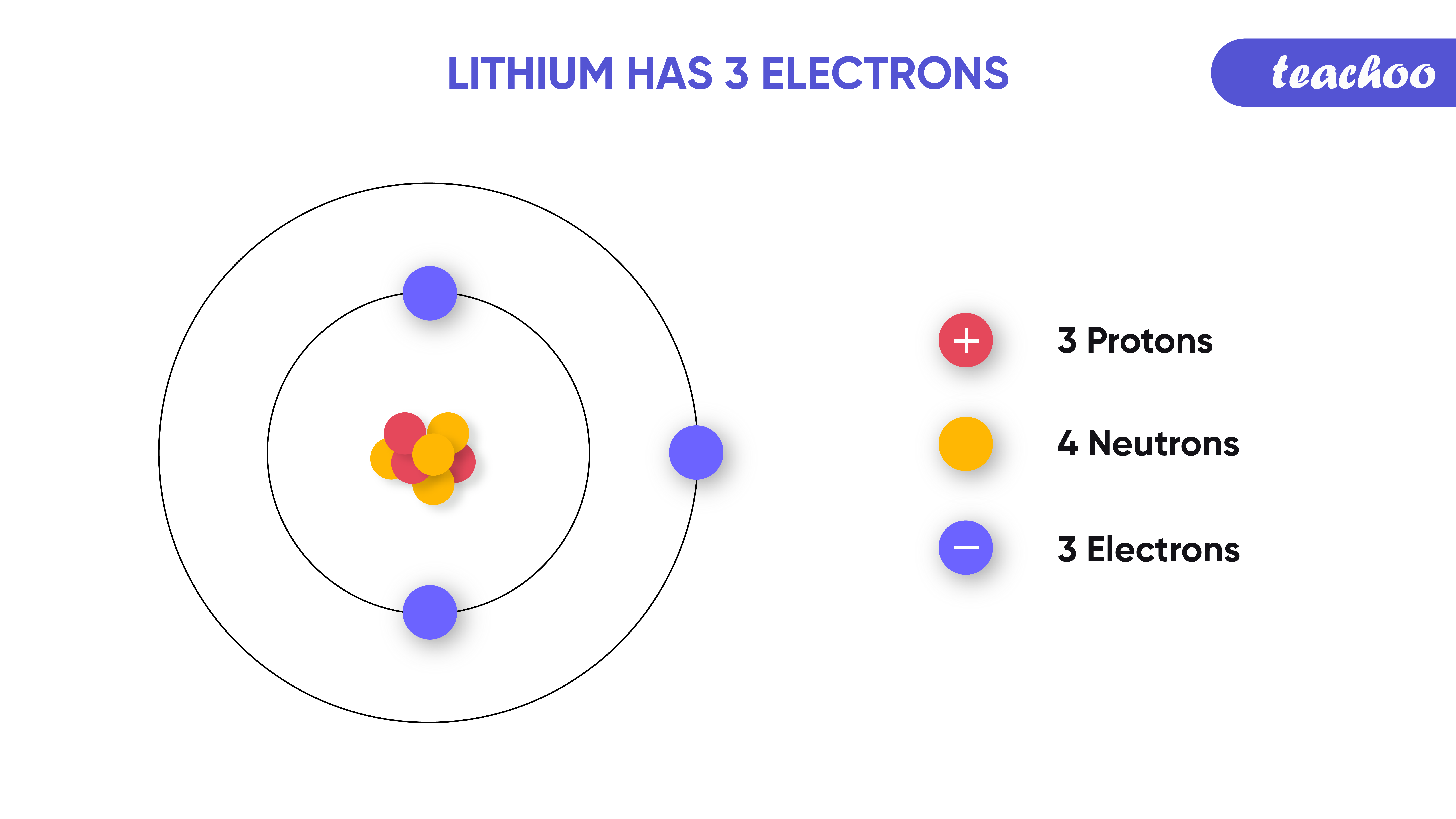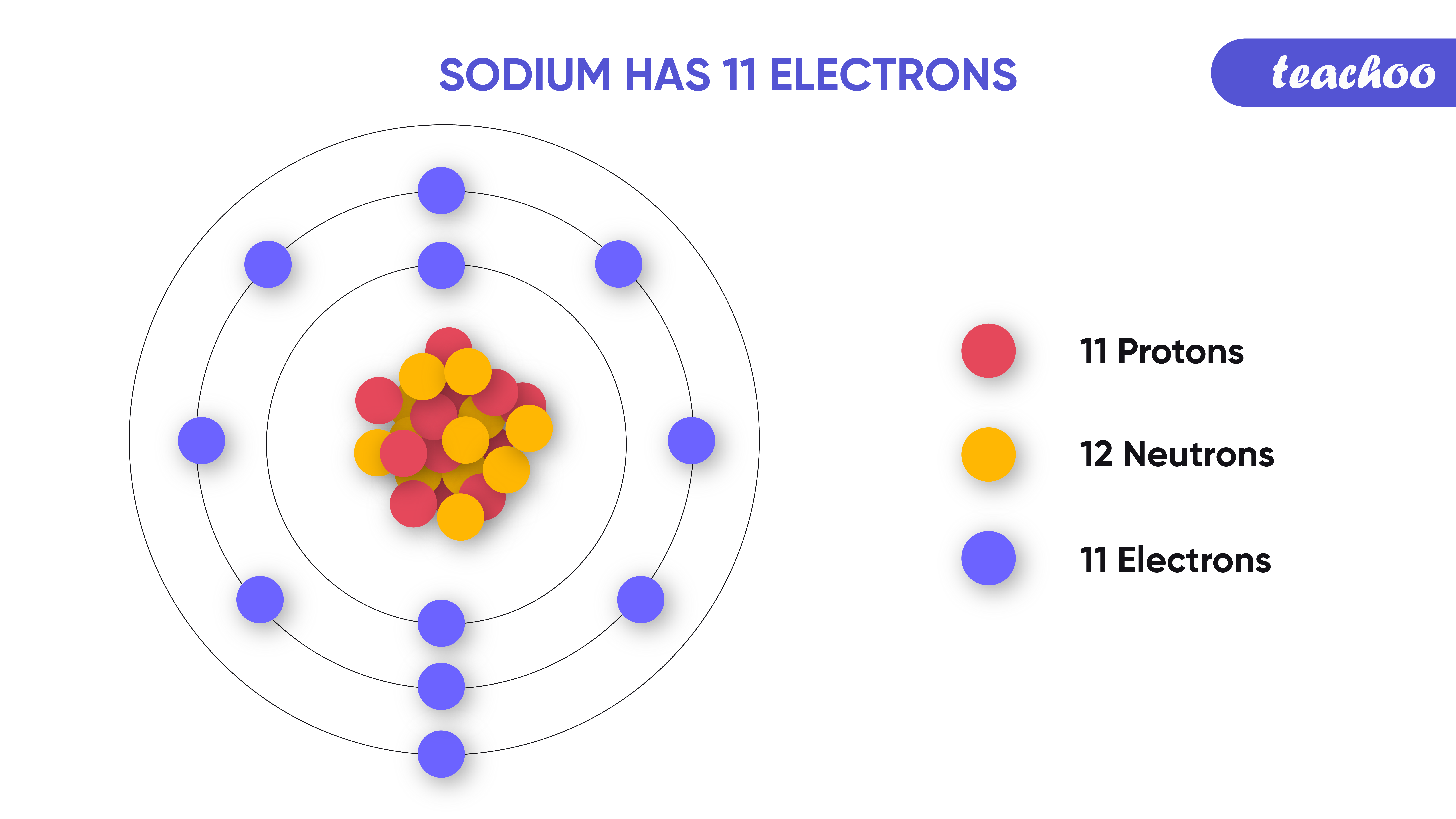Concepts

Class 9
Chapter 4 Class 9 - Structure of Atom

We know that as per Bohr Model.

• Electrons move around Nucleus of an atom in discrete orbits .
• These are also called energy levels or shells .

What is the number of Electrons in a Shell?

• The distribution of the electrons in the energy shells is known as electronic configuration .

• This is based on the Bohr-Bury scheme according to which, the maximum number of electrons that can be present in a particular energy shell of an atom is given by 2n 2 , where “n” is the number of energy shells.

• The energy shells K, L, M, N are the first four energy shells given by Bohr .

• So, the maximum number of electrons which can be accommodate in each shell is given as:
 Electron Shell Maximum Capacity K shell 2 electron L shell 8 electron M shell 18 electron N shell 32 electronNote: - The order of energy of these energy shells starting form nucleus is K < L < M < N.

## How is Maximum Number of Electrons in a shell Calculated

It is calculated by using formula, 2n 2

Example: -

• For K shell, n = 1

So number of Electrons = 2n 2 = 2 x 1 2 = 2

• For L shell, n = 2

So number of Electrons = 2n 2 = 2 x 2 2 = 2 x 4 = 8

• For M shell, n = 3

So number of Electrons = 2n 2 = 2 x 3 2 = 2 x 9 = 18

• For N shell, n = 4

So number of Electrons = 2n 2 = 2 x 4 2 = 2 x 16 = 32

Note:   Electrons are first filled in the innermost shell first.

Then, it is filled in the outer shell.

Example:

1. Helium has 2 Electrons
All these are in K Shell (as K Shell can hold max 2 electrons)2. Lithium has 3 Electrons
2 of these are held in K Shell (as K Shell can hold max 2 electrons)
Remaining 1 are held in L Shell3. Sodium has 11 Electrons
2 of this are held in K Shell (as K Shell can hold max 2 electrons)
8 of this are held in L Shell (as L Shell can hold max 8 electrons)
Remaining 1 is held in M ShellExamples -

In text Question - Page 50 Q1, Q2

NCERT Back Exercise - Q6, Q9, Q12

Introducing your new favourite teacher - Teachoo Black, at only ₹83 per month